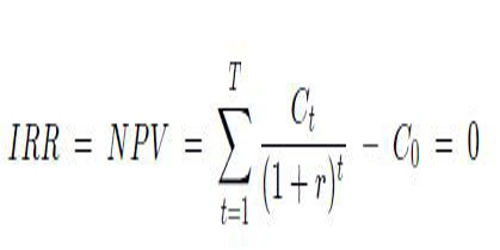Pooled Internal Rate of Return (PIRR) is a method of determining the internal rate of returns (IRR) for a group of funds, as opposed to calculating an average rate of return across the funds. PIRR is generally considered a more accurate calculation than an average rate of return; the overall IRR of the portfolio can then be calculated from this pool of cash flows.

The pooled internal rate of return can be expressed as a formula:Where:

IRR = internal rate of return

NPV = net present value

Ct= the pooled cash flows expected at time t

r = the risk-free rate of return

With the help of pooled internal rate of return (PIRR), the collective rate of return of a single portfolio is calculated by using different cash flows of different projects of the single portfolio. The cash flows that are used for calculating the pooled internal rate of return are actually the individual cash flows of each project. A pooled cash flow is created with the help of individual cash flows and the internal rate of return is then calculated with the help of this pooled cash flow.

The best usage of the pooled internal rate of return and the calculation of the pooled cash flow is in the case of private equity that has a number of funds and it is important to calculate pooled cash flows. With the help of the pooled internal rate of return, we can have an idea of the overall internal rate of all the groups of the equity. Another useful advantage of pooled internal rate of return is that it can help in calculating the average rate of return of the equity. Thus the pooled internal rate of return gives a better picture of the overall performance of an entity as compared to the average IRR.

The internal rate of return (IRR) is a metric used in capital budgeting to estimate the profitability of potential investments. The internal rate of return is a discount rate that makes the net present value (NPV) of all cash flows from a particular project equal to zero. IRR calculations rely on the same formula as NPV does. The pooled IRR is the rate of return at which the discounted cash flows (the net present value) of all projects in the aggregate are equal to zero.

The pooled internal rate of return (PIRR) can be used to find the overall rate of return for an entity running multiple projects or for a portfolio of funds each producing their own rate of return. The pooled IRR concept can be applied, for example, in the case of a private equity group that has several funds. The pooled IRR can establish the overall IRR for the private equity group and is better suited for this purpose than say average IRR of the funds, which may not give an accurate picture of overall performance.

Information Sources: